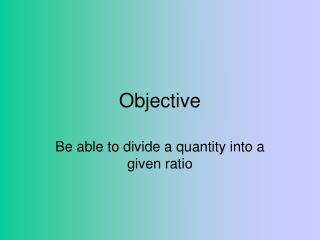Download PresentationObjective

# Objective - PowerPoint PPT PresentationDownload Presentation## Objective

- - - - - - - - - - - - - - - - - - - - - - - - - - - E N D - - - - - - - - - - - - - - - - - - - - - - - - - - -
##### Presentation Transcript

1. Objective Be able to divide a quantity into a given ratio

2. Ratio: What does this mean? Ratio is used to compare the sizes of two (or more) quantities. For example: a drink is made by mixing two parts orange juice with five parts water. This relationship of 2 to 5 can be written as the ratio 2:5. So the ratio of iron to copper is 60:40. We could simplify this to give 3:2 A steel alloy rod is made up of 60% iron and 40% copper.

3. Dividing a quantity in to a ratio Divide 560kg in the ratio 3:4 Total parts 1 part parts parts 3 + 4 = 7 560  7 = 80 3 3 x 80 = 240kg 4 4 x 80 = 320kg Divide 560kg in the ratio 3:4 is 240kg:320kg

4. Time to try some! £400 : £500 £75 : £125 £245 : £385 840kg : 1365kg 125h : 150h : 175h 125lbs : 200lbs : 225lbs • £900 into the ratio 4:5 • £200 into the ratio 3:5 • £630 into the ratio 7:11 • 2205kg into the ratio 8:13 • 450 hours into the ratio 5:6:7 • 550 lbs into the ratio 5:8:9